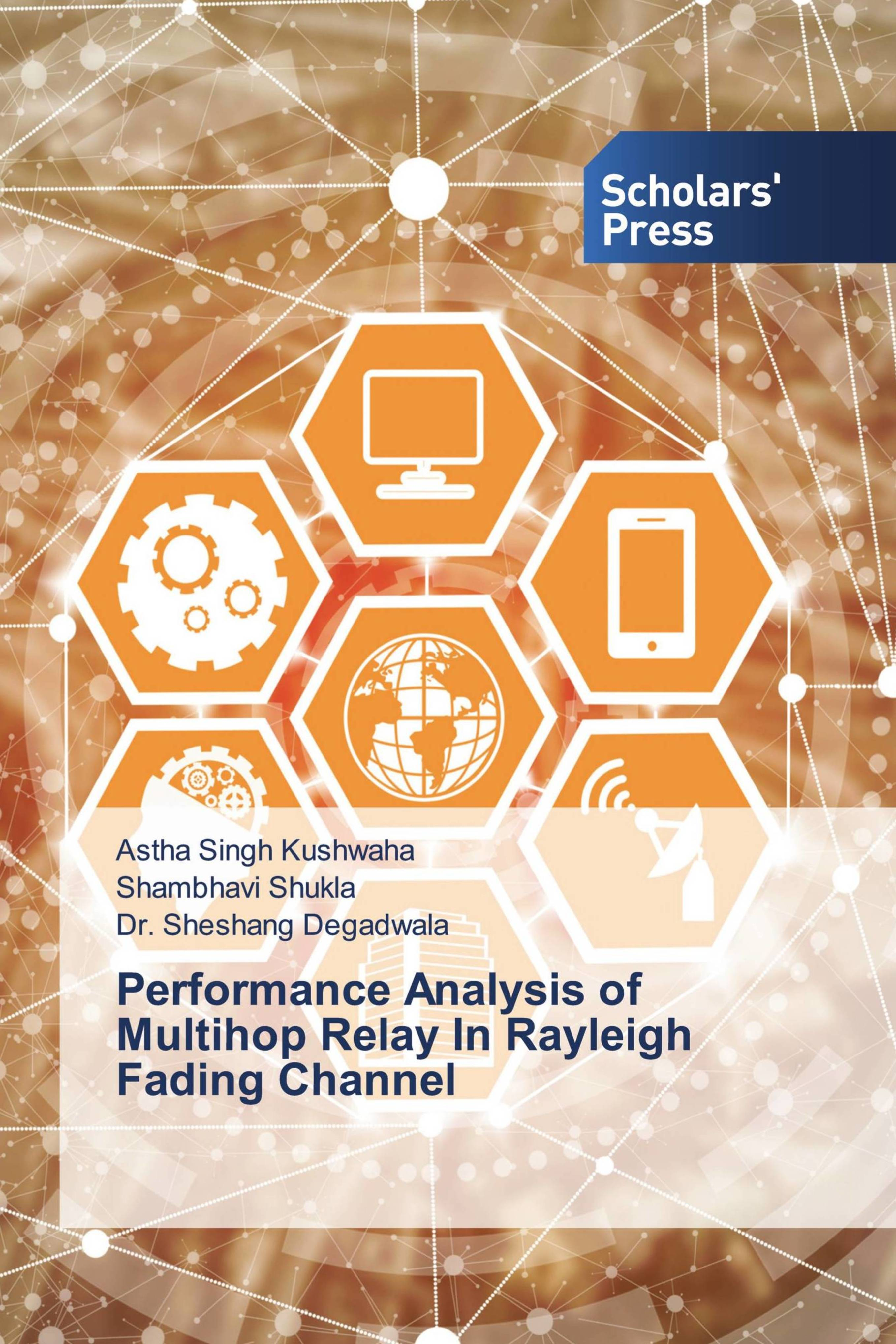• library@msu.ac.th
• Academic Resource Center Mahasarakham University
• library@msu.ac.th
• Academic Resource Center Mahasarakham Universityหมวดหมู่### Performance Analysis Of Multihop Relay In Rayleigh Fading Channel

There is always a need to develop more efficient wireless communication systems for higher data rates. The performance analysis of Bit error rate (BER) of two combining schemes Maximal ration combining (MRC) and Selection Combining (SC) techniques. In this contribution all the study is based on MATLAB environment when communication over Rayleigh fading channels. A simple BPSK modulation is considered. Radio wave propagation through wireless channels is always being a complicated phenomenon which is attributed to various effects such as multipath and shadowing. An exact mathematical illustration of this phenomenon is either unknown or too complex to model for communication systems analyses. Therefore, to analyze such scenario statistical approach is considered. So, immense efforts have been devoted to the statistical modeling and characterization of these different effects.

ใส่ตะกร้า
• ISBN9783330651876
• ประเภท E-Book
• ผู้แต่ง Astha Singh Kushwaha,Shambhavi Shukla and Dr. Sheshang Degadwala
• สำนักพิมพ์ Scholars' Press
• ครั้งที่พิมพ์ 1
• ปีที่พิมพ์2022
• ภาษาภาษาอังกฤษ
• หมวดหมู่คอมพิวเตอร์และเทคโนโลยี
###### : ข้อมูลหนังสือ

There is always a need to develop more efficient wireless communication systems for higher data rates. The performance analysis of Bit error rate (BER) of two combining schemes Maximal ration combining (MRC) and Selection Combining (SC) techniques. In this contribution all the study is based on MATLAB environment when communication over Rayleigh fading channels. A simple BPSK modulation is considered. Radio wave propagation through wireless channels is always being a complicated phenomenon which is attributed to various effects such as multipath and shadowing. An exact mathematical illustration of this phenomenon is either unknown or too complex to model for communication systems analyses. Therefore, to analyze such scenario statistical approach is considered. So, immense efforts have been devoted to the statistical modeling and characterization of these different effects.